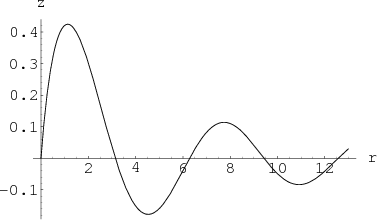# Math Insight

### Image: Plot of a decaying sinusoidPlot of the decaying sinusoidal function $f(r)=\sin r/(r+1)$.

Image file: plot_decay_sine.png